## RS Aggarwal Class 8 Solutions Chapter 2 Exponents Ex 2C

These Solutions are part of RS Aggarwal Solutions Class 8. Here we have given RS Aggarwal Solutions Class 8 Chapter 2 Exponents Ex 2C.

Other Exercises

Objective Questions :
Tick the correct answer in each of the following:

Question 1.
Solution:Question 2.
Solution: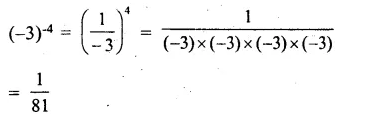Question 3.
Solution: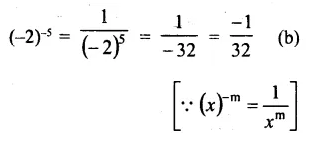Question 4.
Solution: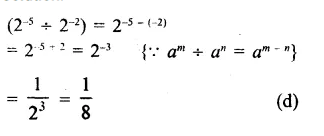Question 5.
Solution:Question 6.
Solution: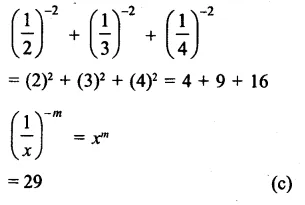Question 7.
Solution: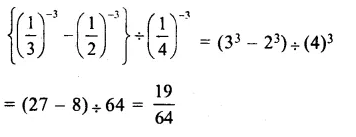Question 8.
Solution: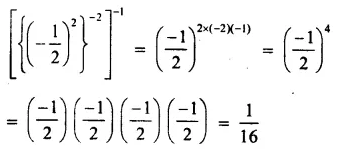Question 9.
Solution: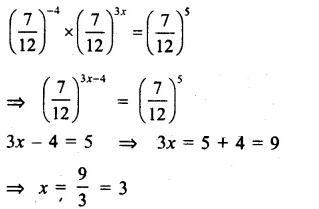Question 10.
Solution:Question 11.
Solution: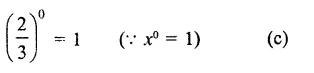Question 12.
Solution: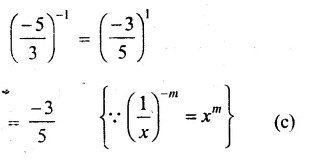Question 13.
Solution: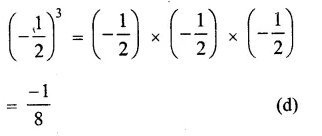Question 14.
Solution: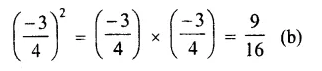Question 15.
Solution:
3670000 = 3.670000 x 1000000
= 3.67 x 106 (c)

Question 16.
Solution: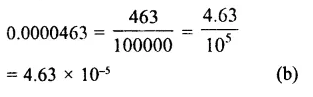Question 17.
Solution:Hope given RS Aggarwal Solutions Class 8 Chapter 2 Exponents Ex 2C are helpful to complete your math homework.

If you have any doubts, please comment below. Learn Insta try to provide online math tutoring for you.